Search Algorithms Sequential Search Linear Search Binary Search

• Slides: 24Search Algorithms Sequential Search (Linear Search) Binary Search Rizwan Rehman Centre for Computer Studies Dibrugarh University 1Searching Arrays Searching is the process of looking for a specific element in an array; for example, discovering whether a certain score is included in a list of scores. Searching, like sorting, is a common task in computer programming. There are many algorithms and data structures devoted to searching. In this section, two commonly used approaches are discussed, linear search and binary search. 2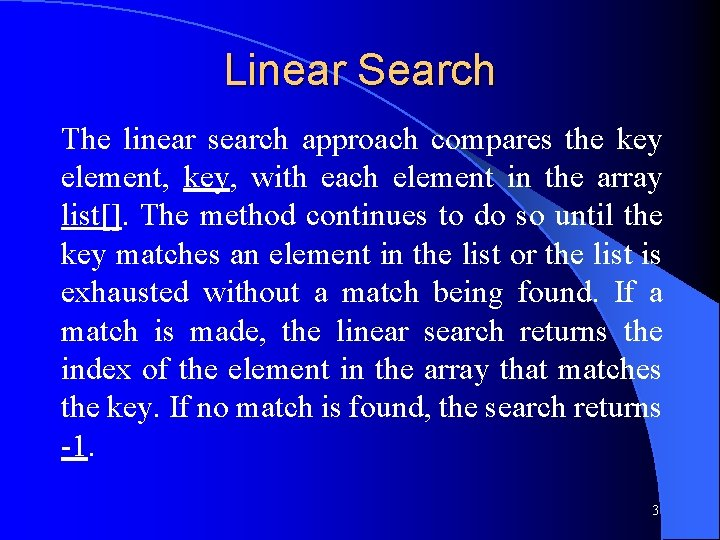Linear Search The linear search approach compares the key element, key, with each element in the array list[]. The method continues to do so until the key matches an element in the list or the list is exhausted without a match being found. If a match is made, the linear search returns the index of the element in the array that matches the key. If no match is found, the search returns -1. 3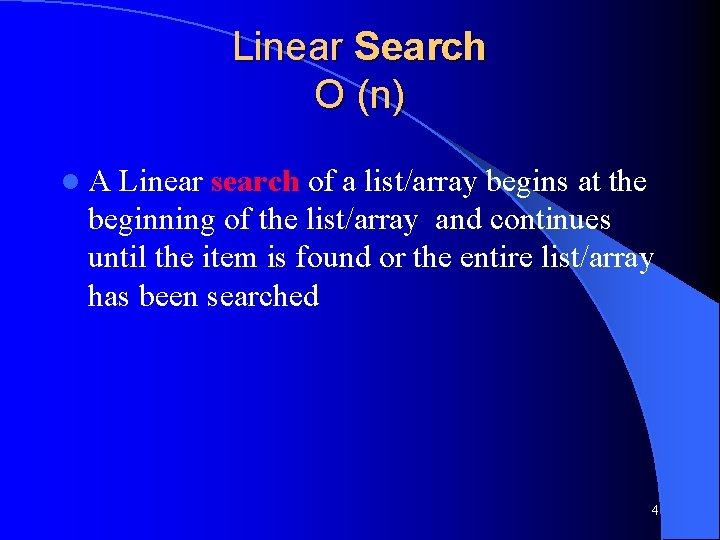Linear Search O (n) l. A Linear search of a list/array begins at the beginning of the list/array and continues until the item is found or the entire list/array has been searched 4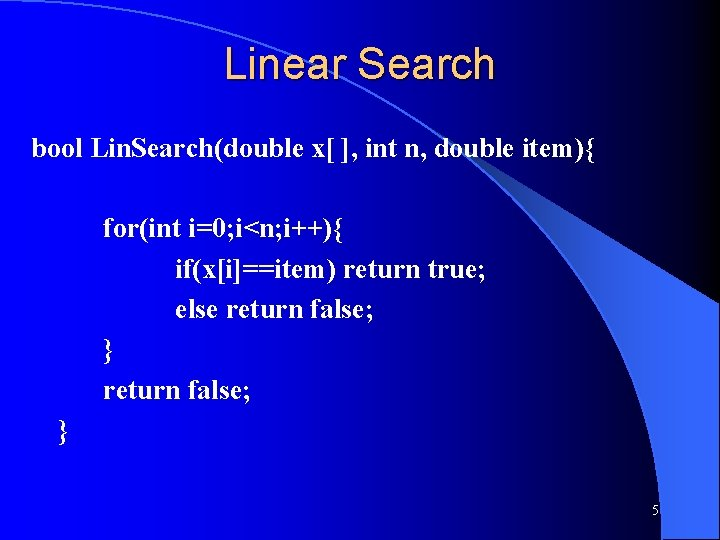Linear Search bool Lin. Search(double x[ ], int n, double item){ for(int i=0; i<n; i++){ if(x[i]==item) return true; else return false; } 5Search Algorithms Suppose that there are n elements in the array. The following expression gives the average number of comparisons: It is known that Therefore, the following expression gives the average number of comparisons made by the Linear search in the successful case: 6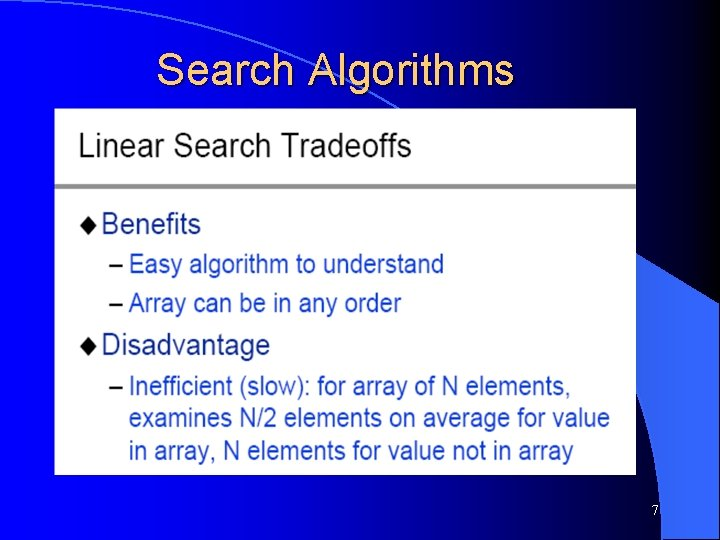Search Algorithms 7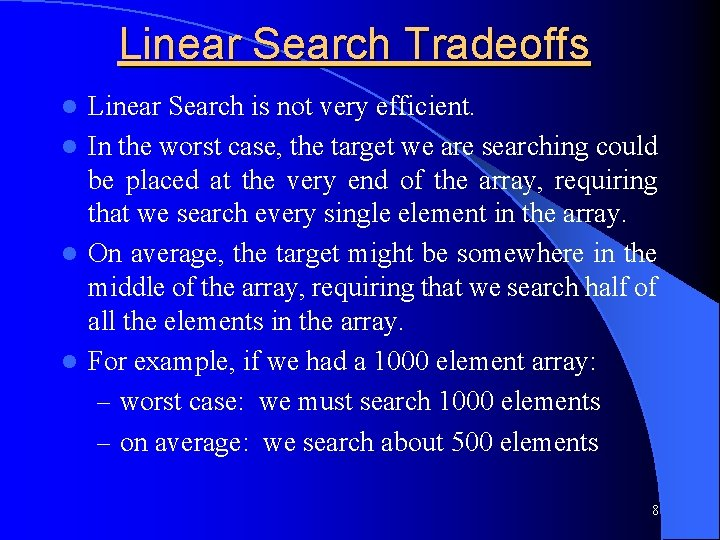Linear Search Tradeoffs Linear Search is not very efficient. l In the worst case, the target we are searching could be placed at the very end of the array, requiring that we search every single element in the array. l On average, the target might be somewhere in the middle of the array, requiring that we search half of all the elements in the array. l For example, if we had a 1000 element array: – worst case: we must search 1000 elements – on average: we search about 500 elements l 8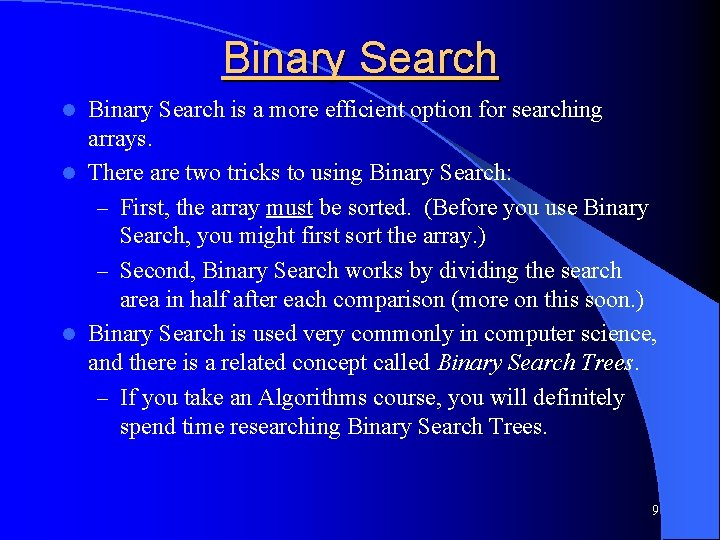Binary Search is a more efficient option for searching arrays. l There are two tricks to using Binary Search: – First, the array must be sorted. (Before you use Binary Search, you might first sort the array. ) – Second, Binary Search works by dividing the search area in half after each comparison (more on this soon. ) l Binary Search is used very commonly in computer science, and there is a related concept called Binary Search Trees. – If you take an Algorithms course, you will definitely spend time researching Binary Search Trees. l 9Binary Search O(log 2 n) l. A binary search looks for an item in a list using a divide-andconquer strategy 10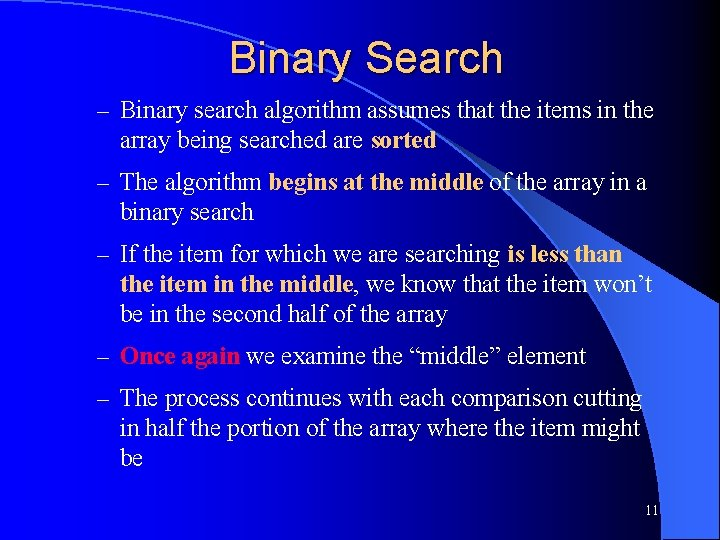Binary Search – Binary search algorithm assumes that the items in the array being searched are sorted – The algorithm begins at the middle of the array in a binary search – If the item for which we are searching is less than the item in the middle, we know that the item won’t be in the second half of the array – Once again we examine the “middle” element – The process continues with each comparison cutting in half the portion of the array where the item might be 11Binary Search 12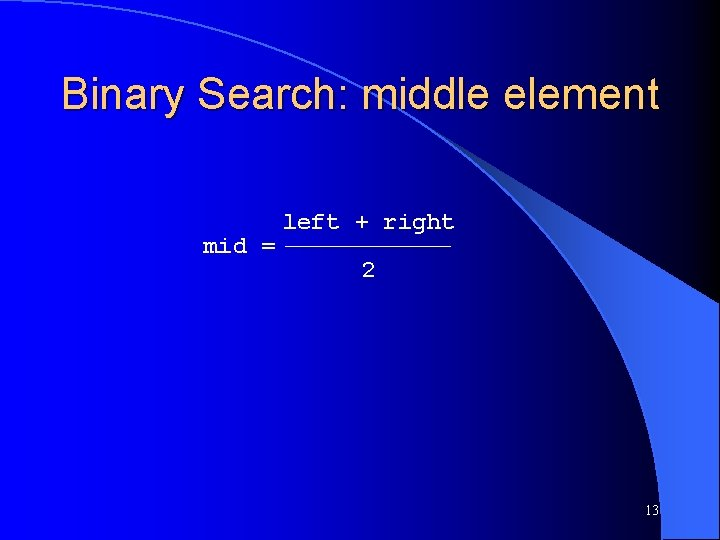Binary Search: middle element mid = left + right 2 13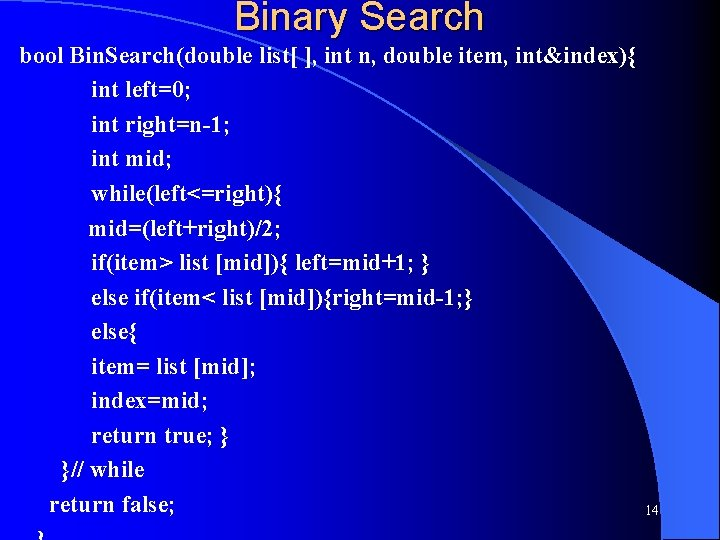Binary Search bool Bin. Search(double list[ ], int n, double item, int&index){ int left=0; int right=n-1; int mid; while(left<=right){ mid=(left+right)/2; if(item> list [mid]){ left=mid+1; } else if(item< list [mid]){right=mid-1; } else{ item= list [mid]; index=mid; return true; } }// while return false; 14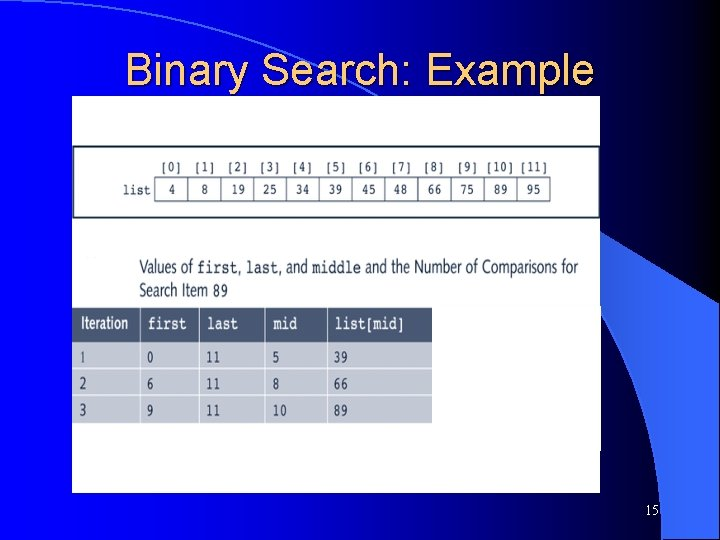Binary Search: Example 15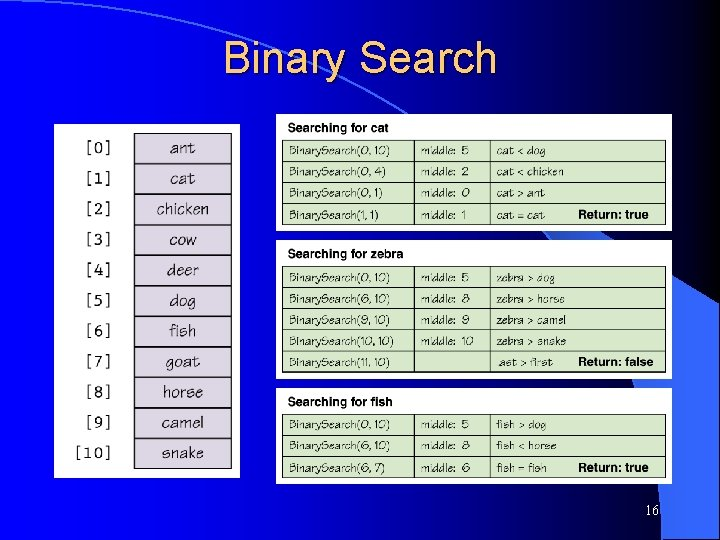Binary Search 16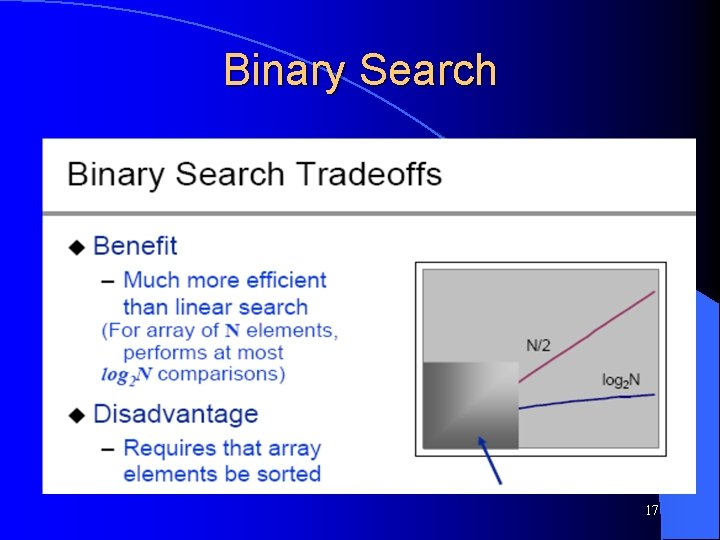Binary Search 17Binary Search Tree 18Binary Search Tree O(log 2 n) bool search(Node * root, int id) { bool found = false; if(root == NULL) return false; if(root->data == id) { cout<<" The node is found "<<endl; found = true; } if(!found) found = search(root->left, id); if(!found) found = search(root->right, id); return found; } 19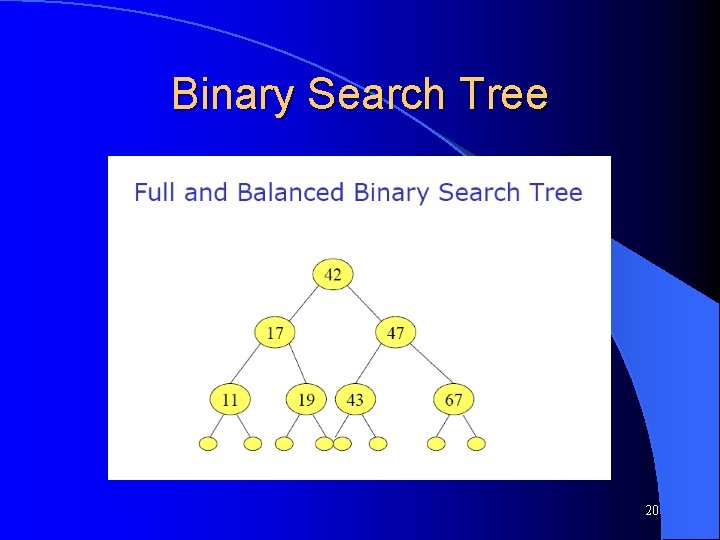Binary Search Tree 20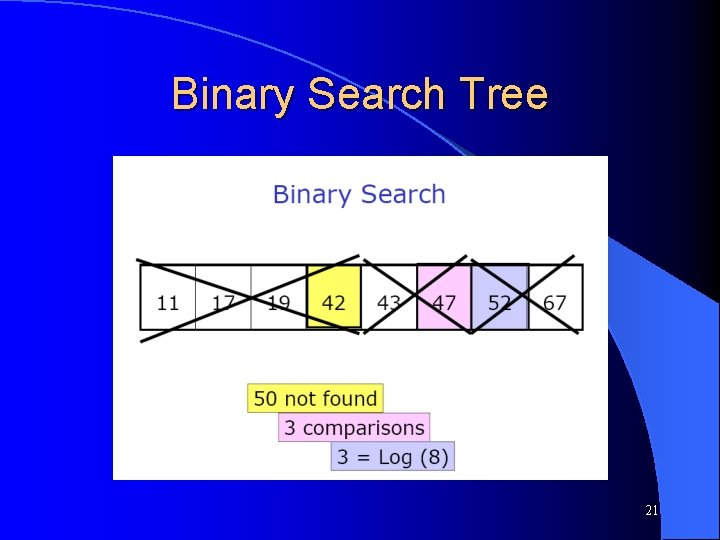Binary Search Tree 21Binary Search Tree 22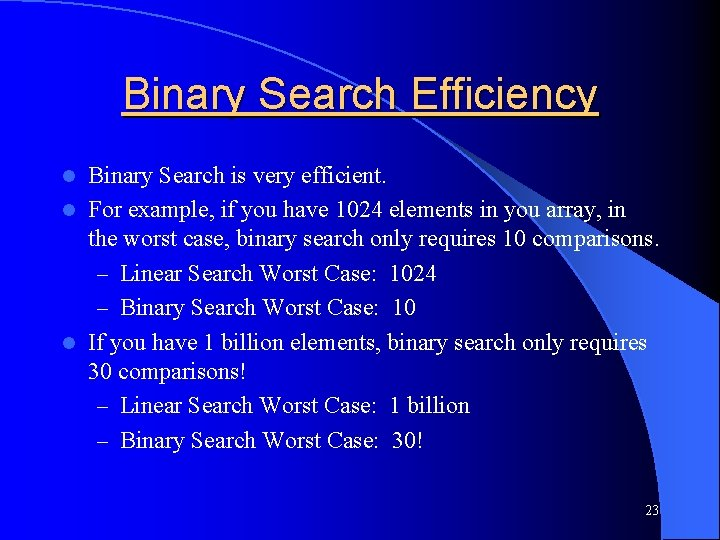Binary Search Efficiency Binary Search is very efficient. l For example, if you have 1024 elements in you array, in the worst case, binary search only requires 10 comparisons. – Linear Search Worst Case: 1024 – Binary Search Worst Case: 10 l If you have 1 billion elements, binary search only requires 30 comparisons! – Linear Search Worst Case: 1 billion – Binary Search Worst Case: 30! l 23Thank You 24